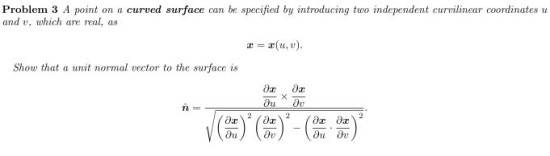# Normal vector of curved surface

## Homework Statement## The Attempt at a Solution

I can understand it intuitively, but can't prove mathematically...Can you help me??

Ray Vickson
Homework Helper
Dearly Missed

## Homework Statement## The Attempt at a Solution

I can understand it intuitively, but can't prove mathematically...Can you help me??

PF rules forbid us from offering help until you have demonstrated an effort and shown your work.

I'm so sorry. It was my first time to write in this forum. I know that cross product makes perpendicular vectors. But in this problem, I don't understand how we explain three dimension by using two parameter, u and v. I searched in internet and thought it is related to gradient. Is it right?

I'm so sorry. It was my first time to write in this forum. I know that cross product makes perpendicular vectors. But in this problem, I don't understand how we explain three dimension by using two parameter, u and v. I searched in internet and thought it is related to gradient. Is it right?

If you use 3 parameters to parametrize a region of space in ℝ3, what you get is a region covering some volume.
However, if you only use 2 parameters to parametrize a region of space in ℝ3, you get a "2D" curved surface located in that 3D space.

Ahh, I got what 2 parameters mean. Thanks. then could you help me more to solve that problem??

Dick
Homework Helper
Ahh, I got what 2 parameters mean. Thanks. then could you help me more to solve that problem??

You know that the partial derivatives are tangent vectors, right? And you know that the cross product is orthogonal to the two vectors, I hope. And dot products are related to cosines of the included angle and cross products are related to sine? Put all of those ingredients together.

I can explain that in word and can image in mind, but i can't in numerical expression... That's my problem...

Dick
Homework Helper
I can explain that in word and can image in mind, but i can't in numerical expression... That's my problem...

Look at the denominator in terms of the included angle ##\theta##. Use ##1-\cos^2(\theta)=\sin^2(\theta)##. Now does that help?

I was so silly. It helps me a lot. Thanks for your help!!

Dick